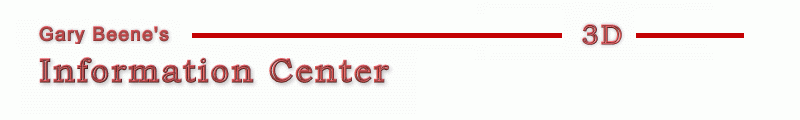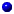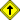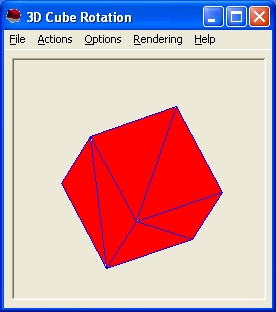Introduction
Overview
History
Books
Tutorials

3D on the Web
Pure Java
Java3D
Flash
VRML
Other

Source Code - VB
Any of today's programming languages can be used to generate 3D images of varying degrees of performance and quality. Visual Basic, either alone or augmented with the use of various API, is just one language option. Throughout this page I'll be introducing and annotating the code to create a 3D rotating cube (wireframe and shaded) using Visual Basic. I'm working on a similar page using Java, primarily because VB cannot be used within a web page, whereas Java can. The completed 3D cube project is called gbCube (7 April 2004). and is available for download. This file is a work in progress, so check regularly for updates.3D Cube - VB Code with APIPure Code (API Replacement)Return to top of document

### 3D Cube - VB Code with API

This section examines the source code of gbCube, a VB application which creates a rotating 3D cube Visual Basic statements, along with an API call for shading (coloring) the faces of the cube. The main form used in gbCube displays a single picturebox, in which the 3D cube is drawn. A variety of buttons, checkboxes, and textboxes are used to allow the user to control the animation or to see various information about the calculations being performed.As was noted in the 3D math page at this site, standard trigonometric calculations or matrix operations can be used to perform the calculations needed to animate a 3D scene. In the example that follows matrices are not used. Code examples of matrix math are, however, provided elsewhere at this site.

The completed 3D cube project is available here (7 April 2004). This file is a work in progress, so check regularly for updates.

The various calculations to be demonstrated, collectively known as the 3D graphics pipeline, include:

• Modelling
• Rotation
• Depth Sorting (Painter's Algorithm)
• Backface culling
• Projection

Modelling (Declarations)
To be consistent with discussions elsewhere on this site, the cube will be modelled using triangles. Twelve triangles are needed, 2 for each of the 6 cube faces. The cube model requires only 8 points, with points shared by multiple triangles.

User-defined types Point and Triangle are defined, with an array P of 8 points and an array T of 12 triangles used to hold the modelling data. The Point UDT declaration is:

```Private Type Point
x As Single
y As Single
z As Single
xp As Single
yp As Single
End Type
```

x, y, and z are the coordinates of the point. xp and yp are the projections of those points onto the computer screen.

The Triangle UDT declaration is:

```Private Type Triangle
p1 As Long
p2 As Long
p3 As Long
ZDepth As Single
DotProduct As Single
End Type
```

p1, p2, and p3 are the points which make up the triangle. Their values are 1-8, corresponding to the array of points already discussed. The ZDepth is the average z-value of the three points. The DotProduct is the value of the dot product between a normal to the plane (calculated use the cross product) and the vector to the point of view. In this example, the point of view is located at coordinates (0,0,POV). The actual value of POV is modified to maintain a constant image whenever the form is resized.

Five global variables are also used. The declaration for those variables is as follows:

```Dim P(8) As Point
Dim T(12) As Triangle
Dim Offset As Single
Dim POV As Single
Dim DeltaTheta As Single
```

• P() - an array of 8 points
• T() - an array of 12 triangles
• Offset - translation of all points needed to center the cube in the computer screen
• DeltaTheta - incremental angle of rotation
• POV - distance from the origin to the point of view

On program startup, a subroutine called Initialization runs, which sets the coordinate values for the points and assigns points to each vertex of all triangles. Values for DeltaTheta, Offset, and POV are also assigned. Both Offset and POV are modified to maintain a centered image whenever the main form is resized.

```Sub Initialize(L As Single)
DeltaTheta = 0.02
POV = 5000   '5000
Timer1.Interval = 25
'points
P(1).x = -L: P(1).y = -L: P(1).z = -L
P(2).x = -L: P(2).y = L: P(2).z = -L
P(3).x = L: P(3).y = L: P(3).z = -L
P(4).x = L: P(4).y = -L: P(4).z = -L
P(5).x = -L: P(5).y = -L: P(5).z = L
P(6).x = -L: P(6).y = L: P(6).z = L
P(7).x = L: P(7).y = L: P(7).z = L
P(8).x = L: P(8).y = -L: P(8).z = L
'triangles
T(1).p1 = 1: T(1).p2 = 4: T(1).p3 = 3
T(2).p1 = 1: T(2).p2 = 3: T(2).p3 = 2
T(3).p1 = 5: T(3).p2 = 1: T(3).p3 = 2
T(4).p1 = 5: T(4).p2 = 2: T(4).p3 = 6
T(5).p1 = 8: T(5).p2 = 5: T(5).p3 = 6
T(6).p1 = 8: T(6).p2 = 6: T(6).p3 = 7
T(7).p1 = 4: T(7).p2 = 8: T(7).p3 = 7
T(8).p1 = 4: T(8).p2 = 7: T(8).p3 = 3
T(9).p1 = 3: T(9).p2 = 7: T(9).p3 = 6
T(10).p1 = 3: T(10).p2 = 6: T(10).p3 = 2
T(11).p1 = 4: T(11).p2 = 1: T(11).p3 = 5
T(12).p1 = 4: T(12).p2 = 5: T(12).p3 = 8
End Sub
```

An alternate approach could have been used to define each triangle. A single Triangle UDT could have been used that contains the coordinates for each point of each triangle vertex. This would have resulted in defining 72 total coordinates, with duplicate entries for vertices shared by triangles.

gbCube uses the array of 8 points (24 coordinates) simply because it reduces the number of calculations by a factor of 3. There is some slight penalty in complexity of the code.

Finally the shading of the triangles the comprise the cube are accomplished using a Windows API called FloodFill. The declaration statement for that API is as follows:

```Private Declare Function FloodFill Lib "gdi32" (ByVal hdc As Long, _
ByVal x As Long, ByVal y As Long, ByVal crcolor As Long) As Long
```

Program Operational Overview
Animation of the cube is achieved by using a timer control with an interval of 50 ms. In each timer event a subroutine called PipeLine is run. Pipeline calls the subroutines for each step required for rendering the 3D cube (the graphics pipeline).

The timer code is as follows:

```Private Sub Timer1_Timer()
PipeLine
End Sub
```

The timer event simply calls the Pipeline subroutine which in turn calls the individual routines that implement the 3D graphics pipeline. All of the individual routines are listed and discussed below.

During load of gbCube, the point and triangle data are initialized as discussed above.

gbCube can display a wireframe version of the cube as well as a shaded (colored) version.

A few words about graphics methods in VB are also in order. gbCube uses VB's built-in line drawing function. It's very fast and simple to use. The line drawing function is used to draw the edges of the cube. gbCube draws the edges in blue and shades the face red. This helps visually resolve the 3D cube features.

Unfortunately VB has no built-in capability to shade (color) an irregular area of the screen. VB does have a routine for coloring rectangles but has none for coloring irregular shapes such as triangles. There are various Windows API which can be used to fill in areas of the screen. The FloodFill API is used by gbCube (see the last section of this page for a pure-VB rendering solution).

The shading used by gbCube is called flat shading - all the pixels of a triangle are colored exactly the same. This approach is simple to use and very fast but is not very realistic. It does not provide color gradients nor does it take into account shadows resulting from the 3D scene's light source. Future enhancements to gbCube will include a light source and more advanced shading algorithms, such as Phon shading.

Here's the Pipeline subroutine that is called in the timer event. It called out a 5-step 3D graphics pipeline. As was just noted, lighting and photo-realistic rendering steps are not included.

```Sub PipeLine()
RotateCoordinatesX DeltaTheta
RotateCoordinatesY DeltaTheta
RotateCoordinatesZ DeltaTheta
If mnuPainter.Checked = True Then SortByZDepth
If mnuBackFace.Checked = True Then BackFaceCulling
If mnuProjection.Checked = True Then CalculatePointProjections
DrawCube
End Sub
```
The If statements correspond to optional execution of the SortByZDepth (Painter's Algorithm), BackFaceCulling, and Projection steps in the 3D graphics pipeline. gbCube was written so that the steps could be turned on/off in order to see the results before and after the steps were performed.

RotateCoordinates
The first step in the gbCube 3D graphics pipeline rotates each of the eight points through an angle of rotation. gbCube allows the user to select any combination of x, y, and z rotations - but the angle of rotation is the same for all three. The new position of each point replaces the old value in the point array P. A separate routine is used for rotation about each axis. This allows for selective axis rotation, which gbCube performs when moving the mouse over the picturebox.

SortByZDepth
At the end of the 3D graphics pipeline, the DrawCube subroutine draws the triangles one at a time starting at the top of the triangle array T. The SortByZDepth routine calculates the average z coordinate of each triangle then sorts the triangle array T so that the objects farthest away are drawn first. The z-depth of each triangle is stored in the triangle array T along with the definition of the points that make up each triangle.

This approach is called the Painter's Algorithm and ensures that the nearest objects will be in front of the farthest objects. It works well but has limitations, such as not working well for intersecting triangles. There are variations of the Painter's Algorithm which address these shortcomings but gbCube uses only the sort routine. For a simple cube this approaches works just fine.

Here's the SortByZDepth subroutine:

```Sub SortByZDepth()
Dim i As Long, temp As Triangle, j As Long
'calculate average Z of all 3 points in each triangle
For i = 1 To 12
T(i).ZDepth = (P(T(i).p1).z + P(T(i).p2).z + P(T(i).p3).z) / 3
Next i
'sort the point array
For i = 0 To UBound(T) - 1
For j = i + 1 To UBound(T)
If T(i).ZDepth > T(j).ZDepth Then
'swap places
temp = T(i)
T(i) = T(j)
T(j) = temp
End If
Next j
Next i
End Sub
```

The sort algorithm used here is called a bubble sort. It works well enough for a few hundred elements to be sorted, but is not suited for more complex 3D scenes. Other VB sort routines can be written which sort up to a hundred times faster. These will be included in future gbCube updates.

BackFaceCulling
As has been discussed, any triangle pointing away from the point of view cannot be seen - it's on the back side of the 3D scene.

```Function BackFaceCulling()
Dim i As Long
For i = 1 To 12
ComputeCrossProduct i
T(i).DotProduct = ComputeDotProduct
Next i
End Function
```

This function calls out two other routines, ComputeCrossProduct and ComputeDotProduct. The code for these is:

```Sub ComputeCrossProduct(i As Long)
Dim x1 As Single, y1 As Single, z1 As Single
Dim x2 As Single, y2 As Single, z2 As Single
'cross products done on position vectors, not displacement vectors
x1 = P(T(i).p2).x - P(T(i).p1).x
y1 = P(T(i).p2).y - P(T(i).p1).y
z1 = P(T(i).p2).z - P(T(i).p1).z

x2 = P(T(i).p3).x - P(T(i).p1).x
y2 = P(T(i).p3).y - P(T(i).p1).y
z2 = P(T(i).p3).z - P(T(i).p1).z

'T(i) is the triangle
'put resulting vector in P(0)
P(0).x = y1 * z2 - y2 * z1
P(0).y = x2 * z1 - x1 * z2
P(0).z = x1 * y2 - x2 * y1
End Sub
Function ComputeDotProduct()
'uses POV vector 0,0,POV  as x1,y1,z1
'used cross product that was stored in P(0) as x2, y2, z2
ComputeDotProduct = 0 * P(0).x + 0 * P(0).y + POV * P(0).z
End Function
```

ComputeCrossProduct is written as a subroutine and places the resulting cross product vector in the point array P position zero. The rest of gbCube only uses positions 1-8 of the array P so position one was unused and available for storing the cross product vector components. Remember that the cross product returns a vector that is normal to the surface of the triangle, which will then be used in a dot product calculation to determine the direction that the triangle faces.

Another very key point to notice in the source code is that the cross product equations you've seen so far assume that the vector components represent position vectors - with starting points at the origin (0,0,0). To calculate the cross product between two triangle edges you must use the displacement vectors which are calculated by the difference of the starting and ending points of the triangle line segments.

ComputeDotProduct calculates the dot product between the POV vector, which is located at (0,0,POV) in gbCube, and the cross product vector as calculated in ComputeCrossProduct. The value is stored in the triangle array T along with the point information for each triangle.

CalculatePointProjections
Until now all point coordinates have been world coordinates - the position of the 3D cube in space. These point coordinates must now be projected onto the computer screen - onto a 2D surface. The subroutine is as follows:

```Sub CalculatePointProjections()
Dim i As Long
For i = 1 To 8
P(i).xp = P(i).x * POV / (POV + P(i).z) + Offset
P(i).yp = P(i).y * POV / (POV + P(i).z) + Offset
Next i
End Sub
```

Each point of the 3D scene must be displayed on the 2D computer screen. The mapping of the points from the 3D scene to the computer screen is called point projection. There are two general forms of projection, parallel and perspective.

With parallel projection, the x-y coordinates of a 3D point simply map one-to-one to the computer screen. The z coordinates are simply dropped. While very simple to perform, the resulting images do not display realistic images in that objects far away will appear to be the same size as objects close in.

gbCube uses perspective projection, which uses the z dimensions to adjust the 2D images to create more realistic images. With perspective projection, objects farther away will appear smaller in the resulting This simulates real life views of scenes with depth.

DrawCube
The final step in the 3D graphics pipeline used by gbCube is to draw the cube on the computer screen. Drawing a cube consists of drawing the line segments which make up the triangles and then filling the triangles with color. The DrawCube routine performs the task of drawing the edges of the triangles and of filling them color (shading). DrawCube utilizes two other routines DrawLine and FillTriangleAPI for the actual drawing and shading.

When projection is enabled, the points used in the display are the projection points, not the true positions of the points in 3D space.

```Sub DrawCube()
Dim i As Long, Draw As Boolean
txtFaces.Text = ""
PictureBox1.Cls
If mnuBackFace.Checked = True Then Draw = True
For i = 1 To 12
If (Draw = True And T(i).DotProduct > 0) Or Draw = False Then
'           If mnuShade.Checked = True Then FillTriangle T(i).p1, _
T(i).p2, T(i).p3
DrawLine T(i).p1, T(i).p2, vbBlue
DrawLine T(i).p2, T(i).p3, vbBlue
DrawLine T(i).p3, T(i).p1, vbBlue
If mnuShade.Checked = True Then FillTriangleAPI _
T(i).p1, T(i).p2, T(i).p3
txtFaces.Text = txtFaces.Text & i & " "
End If
Next i
End Sub

Sub DrawLine(p1 As Long, p2 As Long, iColor As Long)
Dim x1 As Single, y1 As Single, x2 As Single, y2 As Single
If mnuProjection.Checked = True Then
'calculate screen x-y after projection
x1 = P(p1).x * POV / (POV + P(p1).z) + Offset
y1 = P(p1).y * POV / (POV + P(p1).z) + Offset   '* 0.8
x2 = P(p2).x * POV / (POV + P(p2).z) + Offset
y2 = P(p2).y * POV / (POV + P(p2).z) + Offset   '* 0.8
Else
'screen x-y is same as object x-y plus Offset
x1 = P(p1).x + Offset
y1 = P(p1).y + Offset '   * 0.8
x2 = P(p2).x + Offset
y2 = P(p2).y + Offset '   * 0.8
End If
PictureBox1.Line (x1, y1)-(x2, y2), iColor
End Sub

Sub FillTriangleAPI(p1 As Long, p2 As Long, p3 As Long)
Dim x As Single, y As Single, z As Single
'find point inside the triangle (centroid)
x = (P(p1).x + P(p2).x + P(p3).x) * 0.33333
y = (P(p1).y + P(p2).y + P(p3).y) * 0.33333
z = (P(p1).z + P(p2).z + P(p3).z) * 0.33333
If mnuProjection.Checked = True Then
'calculate screen x-y after projection
x = x * POV / (POV + z) + Offset
y = y * POV / (POV + z) + Offset
Else
'screen x-y is same as object x-y plus Offset
x = x + Offset
y = y + Offset
End If
x = x / Screen.TwipsPerPixelX
y = y / Screen.TwipsPerPixelY
FloodFill PictureBox1.hdc, x, y, vbBlue
End Sub

```

Note that the filling of the triangles is done after the points of the cube are projected onto the computer screen, so the filling routine uses the projection x-y coordinates, not the true coordinates of the 3D cube points.

The FillTriangleAPI subroutine uses the Windows FloodFill API. Starting at a point on the screen the API fills adjacent pixels with the picturebox fillcolor (a property of the picturebox) - spreading until it hits a boundary of a specified color.

For the specified starting point, gbCube uses the centroid of the triangle. The centroid is the intersection of the 3 lines that bisect the 3 angles of the triangle. It's calculation is simply the average of the x, y, and z coordinates. The centroid is always found within the boundary of the triangle - as required for the FloodFill API to fill only the interior of the triangle.Return to top of document

### Pure Code (API Replacement)

VB simply doesn't have capable, built-in functions to support the needs of 3D rendering. It's graphic tools are very simple - limited to points, lines, and a few basic shapes. That's why the code above uses the FillTriangleAPI subroutine to access the Windows API. The result is a much smoother rendering.

However, it is possible use the built-in VB Line function to create a crude equivalent to the FloodFill API. The results are very fast but do not provide 100% pixel shading.

In the non-API approach, a large number of lines are drawn from one point of the triangle to the two other points, distributed along the line segment between the other two points to provide a fan-like coverage of the entire triangle's surface area. The choice of 200 lines to shade each triangle was experimentally observed to give reasonably good pixel coverage but still be within the ability of VB to process within the time intervals used for rotation. The approach is imperfect and does leave some pixels unshaded within the triangles.

The source code for the non-API approach is provided below. The routine is included in the gbCube distribution and can be use by selecting the appropriate context menu item from the picturebox in gbCube.

```Sub FillTriangle(p1 As Long, p2 As Long, p3 As Long)
Dim DeltaX As Single, DeltaY As Single, i As Long
Dim x1 As Single, y1 As Single, x2 As Single, y2 As Single
If chkProjection.Value = vbChecked Then
x1 = P(p1).xp
y1 = P(p1).yp
DeltaX = (P(p3).xp - P(p2).xp) / 200
DeltaY = (P(p3).yp - P(p2).yp) / 200
x2 = P(p2).xp
y2 = P(p2).yp
Else
x1 = P(p1).x + Offset
y1 = P(p1).y + Offset
DeltaX = (P(p3).x - P(p2).x) / 200
DeltaY = (P(p3).y - P(p2).y) / 200
x2 = P(p2).x + Offset
y2 = P(p2).y + Offset
End If
For i = 1 To 200
x2 = x2 + DeltaX
y2 = y2 + DeltaY
PictureBox1.Line (x1, y1)-(x2, y2), vbRed
Next i
End Sub
```